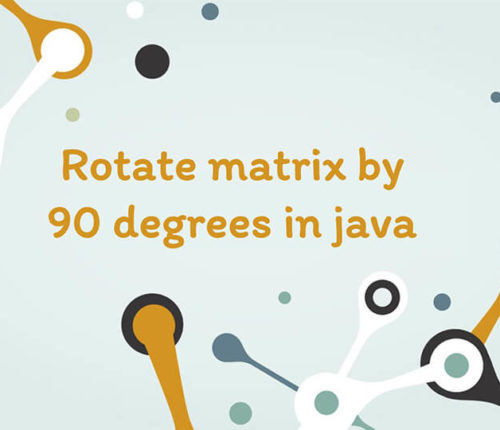•20 May

### Rotate Matrix by 90 degrees in java

In this article, we will look into another interesting problem related to 2D Arrays. Given a Matrix of N X N Dimension we have to Rotate matrix by 90 degrees. We will perform Rotation both Clockwise i.e. Right Rotation and Anti-Clockwise i.e. Left Rotation respectively. We will discuss each operation in detail along with a […]

•09 October

### Java program to find first and last digit of a number

In this article, we are going to find first and last digit of a number in Java. To find first and last digit of any number, we can have several ways like using modulo operator or pow() and log() methods of Math class etc. Let’s see some examples. Before moving to example, let’s first create […]

•30 September

### Find Perfect Number in Java

In this article, we are going to find whether a number is perfect or not using Java. A number is called a perfect number if the sum of its divisors is equal to the number. The sum of divisors excludes the number. There may be several approaches to find the perfect number. For example: 28 […]

•28 September

### Number guessing game in java

In this article, we will implement Number guessing game in java. The number guessing game is based on a concept where player guesses a number between a range. If player guesses the exact number then player wins else player looses the game. Since this game provides limited attempts, so, player must guess the number with […]

•03 October

### Happy Number program in Java

In this article, we are going to learn to find Happy Number using Java. Let’s first understand, what is Happy Number? What is a Happy Number? A number which leaves 1 as a result after a sequence of steps and in each step number is replaced by the sum of squares of its digit. For […]

•30 December

### Rock Paper Scissors Game in Java

Learn about how to implement Rock Paper Scissors game in java.

•28 September

### How to find Magic Number in Java

In this article, we are going to learn to find Magic Number using Java. Let’s first understand, what is Magic Number? What is a Magic Number? A number which leaves 1 as a result after a sequence of steps and in each step number is replaced by the sum of its digits. For example, if […]

•17 March

### PriorityQueue in Java 8

In this post, we will see about Java 8 PriorityQueue. When the objects are supposed to be processed on the basis of their priority, in that scenario we use PriorityQueue. It’s a special type of queue (also, unbound queues) where the elements can be ordered either as per their natural ordering or based on a […]

•05 October

### Print prime numbers from 1 to 100 in java

In this program, we will print prime numbers from 1 to 100 in java. A prime number is a number which has only two divisors 1 and itself. To check if the number is prime or not, we need to see if it has any other factors other than 1 or itself. If it has, […]